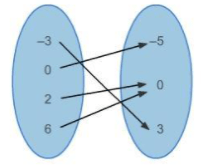# Worksheet on Math Relation | Math Relation Questions with Answers

Students can practice various questions from Relation to get grip on the concepts. This Worksheet on Math Relation covers various topics like Mapping for Relations, Representing Relations using a set of ordered pairs, arrow diagrams, etc. Solve the Ample Problems provided in the Math Relation Worksheet and get a better idea of the concepts within it. Practice the problems on your own and cross-check your solutions with the step by step solutions provided to understand where you went wrong.

1. If A= {3, 4, 5, 6, 7, 8}, B = {9, 10, 11, 12}. What is the number of elements in AxB?

Solution:

Given n(A) = 6

n(B) = 4

n(AxB) = 24

2. Determine the domain and range of the given function

{11, -5), (8, -3), (5, 2), (7, 6), (6, -10)}

Range =_____

Domain = _____

Solution:

Given Function is {11, -5), (8, -3), (5, 2), (7, 6), (6, -10)}

Domain is the first component of the ordered pairs and second component in the ordered pairs is the Range.

Domain = {11, 8, 5, 7, 6}

Range = {-5, -3, 2, 6, -10}

3. Represent Relation {(-3, 3) (0, -5) (2, 0) (6, 0)} using an Arrow Diagram?

Solution:

Take the Domain Values on the left column and Range Values in the Right Column and mark the relation between them using arrows.4. Let A = {10, 15, 18, 21, 24} B = { 3, 5, 6, 7} be two sets and let R be a relation from A to B ‘is multiple of’. Represent in the Set of Ordered Pairs?

Solution:

R = {(10, 5) (15, 3) (18, 3) (18, 6) (21, 3) (21, 7) (24, 6)}

5. Given the relation R = {(3,4), (7,-1), (x,7), (-3,-4)}. Which of the following values for x will make relation R a function?
(a) 8
(b) 7
(c) -3
(d) 3

Solution:

(a) 8

To make relation R a Function we need to have a value that doesn’t repeat with the first components of the ordered pairs. Therefore, among all the options 8 is the value that is unique.

6. State whether the following statements are true or false

(i) All Functions are Relations

(ii) All Relations are Functions

(iii) A relation is a set of input and output values that are related in some way

Solution:

(i) True

(ii) False

(iii) True

7. Express the Relation as a Set of Ordered Pairs?Solution:

Expressing the above Relation as a Set of Ordered Pairs we get

R = {(-4, 0) (-4, 9) (-4, 11)}

8. If A = {u, v, w} and B = {x, y}, find A × B and B × A. Check whether the two products equal or not?

Solution:

Given A = {u, v, w} and B = {x, y}

AxB = {(u,x) (u, y) (v,x) (v,y) (w, x) (w, y)}

BxA = {(x,u) (y, u) (x, v) (y, v) (x, w) (y, w)}

AxB and BxA don’t have the same ordered pairs.

Therefore,  AxB ≠ BxA.

9. If (u/2 + 1, v+2) = (1, 2/5), find the values of u and v?

Solution:

Given (u/2 + 3, v+2) = (1, 2/5)

As per the Equality of Ordered Pairs we have

u/2+3 = 1

u/2 = 1-3

u/2 = -2

u = -2*2

u = -4

v+2 = 2/5

v = 2/5-2

v =(2-4) /5

v = -2/5

Therefore, values of u and v are -4, -2/5.

10. Range is the Set of _____ when it comes to Relations in Math?

Solution:

The range is the set of y-values when it comes to Relations in Math.